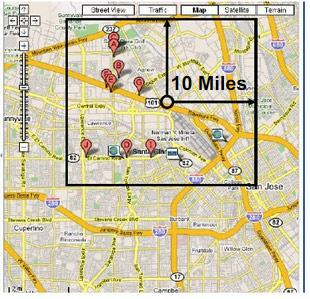# How to find Point of Interests in nearby location of a GPS coordinate?

Global Positioning System- GPS is diversely used nowadays. Google Earth or Google Map is making life easier if someone want to view the road to a particular destination or when someone want to find out nearby hotels if he/she has his/her current GPS location coordinates. As a developer while using API of google map, when someone want to find out nearby places or point of interest within certain radius, then there is two ways which can be implemented. Both ways are about using mysql queries but only difference is the execution time.

If we have find out 10 nearby hotels and sort them by distance; we are provided with: a TABLE having hotel list with hotel name and corresponding GPS coordinates

Table: HOTELS

 Hotel Name Latitude Longitude ABC 28.2354 -78.5874

and the current GPS location (lat, lon).

So, the main task is: how to calculate distance between us and hotels?

We can calculate the distance between two points using The Haversine Formula, which is described as:

For two points on sphere of radius R with latitude ϕ1 and ϕ2, latitude separation Δϕ= ϕ1- ϕ2, and longitude separation Δλ the distance between two points:Where, R= radius of Earth (3965 miles)

Δlat= lat2-lat1; Δlong=long2-long1For MYSQL, this can be written as:

956*2*ASIN( SQRT ( POWER (SIN((orig.lat – dest.lat)*pi()/180 / 2),2) + COS(orig.lat * pi()/180) * COS(dest.lat *pi()/180) * POWER(SIN((orig.lon – dest.lon) *pi()/180 / 2), 2) ) ) as distance

Finally, to find nearby hotels,

set @orig_lat=122.4058;

set @orig_lon=37.7907;

set @dist=10;

SELECT *, 3956 * 2 * ASIN(SQRT( POWER(SIN((@orig_lat – abs( dest.lat)) * pi()/180 / 2),2) + COS(@orig_lat * pi()/180 ) * COS( abs (dest.lat) *  pi()/180) * POWER(SIN((@orig_lon – dest.lon) *  pi()/180 / 2), 2) )) as distanceFROM hotels desthaving distance < @distORDER BY distance limit 10;

RESULT:

| hotel_name | lat | lon | dist ||

Hotel Astori.. | 122.41 | 37.79 | 0.0054 ||

Juliana Hote.. | 122.41 | 37.79 | 0.0069 ||

Orchard Gard.. | 122.41 | 37.79 | 0.0345 ||

Orchard Gard.. | 122.41 | 37.79 | 0.0345 |

|| …

10 rows in set

Though the result is as expected but its execution time is too long for web, in this case its about 4.10 sec which is very slow.

So, is there any fast method to carry the task?

Yes, there is. To speed up the query, when we only need hotels in 10 miles radius, NO NEED TO SCAN THE WHOLE TABLE.Then a next question arises that how to find out the needed coordinates?

We know,

1° of Latitude ~= 69 miles (approx.)

1° of longitude ~= cos(latitude)*69

To calculate lat and lon for the rectangle,

set lat1 = mylat-(dist/69);

set lat2 = mylat+(dist/69);

Now we can modify the previous query as:

SELECT destination.*, 3956 * 2 * ASIN(SQRT( POWER(SIN((orig.lat – dest.lat) *  pi()/180 / 2), 2) +COS(orig.lat * pi()/180) * COS(dest.lat * pi()/180) * POWER(SIN((orig.lon -dest.lon) * pi()/180 / 2), 2) )) as distance FROM user_destination , user_origin WHERE origin.id=userid and destination.longitude between lon1 and lon2 and destination.latitude between lat1 and lat2;

Using MYSQL Stored Procedure:

CREATE PROCEDURE geodist (IN userid int, IN dist int)BEGIN declare mylon double;

declare mylat  double; declare lon1 float; declare lon2 float; declare lat1 float; declare lat2 float;

— get the original lon and lat for the userid

SELECT longitude, latitude into mylon, mylat from users where id=userid limit 1;

— calculate lon and lat for the rectangle:

set lat1 = mylat-(dist/69); set lat2 = mylat+(dist/69);

— run the query:

SELECT destination.*,3956 * 2 * ASIN(SQRT( POWER(SIN((orig.lat -dest.lat) * pi()/180 / 2), 2) +COS(orig.lat * pi()/180) * COS(dest.lat * pi()/180) *POWER(SIN((orig.lon -dest.lon) * pi()/180 / 2), 2) )) as distance FROM users_destination, users_origin WHERE origin.id=userid and destination.longitude between lon1 and lon2 and destination.latitude between lat1 and lat2 having distance < dist ORDER BY Distance limit 10;

END \$\$

SPEED: When these method were tested for US and Canada zip code table which contains 8,00,000 records,

Time take by Original query (full table scan) was 8 sec.

Time taken by Optimized query (Stored Procedure) was 0.06 to 1.2 seconds (depending upon the number of Point of interest /records in the given radius)

So, by using stored procedure method we can speed our query execution time to much extent.

Now, sometime we need to both geo Search and FULL TEXT search, in that case we can perform the query as:

Example – find nearest streets, where name contains “OAK”.

MYSQL query will be as:

SELECT destination.*,3956 * 2 * ASIN(SQRT(POWER(SIN((orig.lat- dest.lat) * pi()/180 / 2), 2) +COS(orig.lat * pi()/180) *COS(dest.lat * pi()/180) *POWER(SIN((orig.lon -dest.lon) * pi()/180 / 2), 2) )) as distance FROM geonames_destination WHERE match(name)against (‘OAK’ in boolean mode) having distance < dist ORDER BY Distance limit 10;

In this way we can find out the nearby points of interest from the current position. This technique is much helpful for developing user interface web application where google map is being integrated.Share On: